• lstm多元时间序列预测
千次阅读
2020-04-08 13:13:09

# 【时间序列预测/分类】 全系列60篇由浅入深的博文汇总：传送门

上篇文章，本文继续介绍用于处理间序列的LSTM模型–多变量LSTM模型（ Multivariate LSTM Models）。

# 2. 多变量 LSTM 模型

多变量（多元）时间序列数据是指每个时间步长有多个观测值的数据。对于多元时间序列数据，有两个常用的模型：

• 多输入序列（Multiple Input Series）
• 多并行序列（Multiple Parallel Series）

## 2.1 多输入序列（Multiple Input Series）

一个问题可能有两个或多个并行输入序列和一个依赖于输入序列的输出序列。输入序列是并行的，每个序列在同一时间步上都有一个观测值。即时间序列预测预测任务，通过以往的数据信息，预测之后某段时间内的数据，比如降雨量预测。在这个任务中，有多个输入序列，比如历史的温度、湿度、光照强度、风速、风向、降雨量等，这些不同类型的数据，我们称之为特征（features），这些特征在相同间隔时间点的采样值构成了并行输入序列。该任务的目的是要使用LSTM模型预测降雨量，那么我们可以使用指定 步长（time steps） 和指定窗口宽度的滑动窗口来提取一小段一小段的序列片段作为训练数据 X i X_i ，这些序列片段最后一行数据对应的降雨量作为期望的预测输出 y i y_i 。那么，一个序列数据片段（一个窗口所截取的数据） X 1 X_1 和一个期望输出 y 1 y_1 就称为一个样本（samples），滑动窗口不断滑动截取数据，可以从原始数据序列中提取多个样本，组成训练数据集。

本文通过由两个并行输入序列和一个输出序列的例子来演示，其中输出序列是输入序列的简单相加。代码实现：

in_seq1 = np.array([10, 20, 30, 40, 50, 60, 70, 80, 90])
in_seq2 = np.array([15, 25, 35, 45, 55, 65, 75, 85, 95])
out_seq = np.array([in_seq1[i]+in_seq2[i] for i in range(len(in_seq1))])
dataset = np.hstack((in_seq1, in_seq2, out_seq))


生成的序列数据为：

[[ 10 15 25]
[ 20 25 45]
[ 30 35 65]
[ 40 45 85]
[ 50 55 105]
[ 60 65 125]
[ 70 75 145]
[ 80 85 165]
[ 90 95 185]]


其实在实际的业务中，基本上都是 txt文件或者csv文件，通过pandas来读取，然后转换成DataFrame结构，再做进一步处理。这样看就比较清晰了，每一列代表一个特征，每一行代表这些特征在不同时间点的采样值。

与单变量时间序列一样，我们必须以输入/输出形式将这些数据构造成样本。LSTM模型通过输入输出的上下文关联来学习输入到输出的映射。LSTM支持单变量或特征的并行输入时间序列。因此，我们需要按照一定的窗口宽度来把数据分成样本，划分过程中要保持两个输入序列的顺序，训练过程中不能shuffle！假设我们选择窗口宽度为3，即通过3个先前时间步长的前两个特征的采样值来预测第三个特征一个时间步长的值，划分样本的滑动步长为1，为了增加文章可读性，代码会在文末的完整代码中给出，此处仅贴出划分结果：

[[10 15]
[20 25]
[30 35]] 65
[[20 25]
[30 35]
[40 45]] 85
[[30 35]
[40 45]
[50 55]] 105
[[40 45]
[50 55]
[60 65]] 125
[[50 55]
[60 65]
[70 75]] 145
[[60 65]
[70 75]
[80 85]] 165
[[70 75]
[80 85]
[90 95]] 185


训练样本和训练标签的shape分别为：

(7, 3, 2) (7,)


数据划分好之后，接下来训练LSTM模型。上一篇文章中提到的任何一种LSTM都可以使用，例如Vanilla、Stacked、Bidirectional、CNN或Conv LSTM模型。下面代码使用普通的（Vanilla）LSTM 定义，通过 input_shape 参数为输入层指定滑动窗口所包含的时间步数（窗口宽度）和并行序列的数目（特征数），其中，sw_width为窗口宽度，经过前文分析，本例中为3，n_features表示特征数目，本例中为2。注意：这里的特征数目是训练数据 X i X_i 所包含的特征数目，所需预测的特征 y y 不包含在内，因此本例中 n_features = 2，而不是3

model = Sequential()


定义完模型，需要使用 fit() 方法训练模型，训练完成之后使用 predict() 方法对新的输入数据进行预测。需要注意的是：在进行预测时，测试数据必须重塑成跟训练数据相同的shape，本例中训练集的shape = (7,3,2) ，因此一个测试数据的shape应该是 (1,3,2)，代码如下：

x_test = array([[80, 85], [90, 95], [100, 105]])
x_test = x_test.reshape((1, sw_width, n_features))
yhat = model.predict(x_test, verbose=0)


## 2.2 多并行序列（Multiple Parallel Series）

交替时间序列问题是存在多个并行时间序列且必须为每个并行时间序列预测一个值的情况。例如，给定上一节中的数据：

[[ 10 15 25]
[ 20 25 45]
[ 30 35 65]
[ 40 45 85]
[ 50 55 105]
[ 60 65 125]
[ 70 75 145]
[ 80 85 165]
[ 90 95 185]]


假设要通过之前的一定时间步长的所有特征的采样值来预测下一个时间步的这些特征的值，这类任务就称为多元预测。假设我们是用之前3个时间步的采样值（滑动窗口宽度为3）作为样本输入数据（X），下一个时间步的采样值作为样本期望输出（y）。那么，可以上以上序列数据划分为：

[[10 15 25]
[20 25 45]
[30 35 65]] [40 45 85]
[[20 25 45]
[30 35 65]
[40 45 85]] [ 50 55 105]
[[ 30 35 65]
[ 40 45 85]
[ 50 55 105]] [ 60 65 125]
[[ 40 45 85]
[ 50 55 105]
[ 60 65 125]] [ 70 75 145]
[[ 50 55 105]
[ 60 65 125]
[ 70 75 145]] [ 80 85 165]
[[ 60 65 125]
[ 70 75 145]
[ 80 85 165]] [ 90 95 185]


这其实是通过函数划分之后输出的结果，具体怎么实现会在下文的代码中给出，先领会样本划分的思想。训练样本和样本标签的shape分别为：

(6, 3, 3) (6, 3)


上一篇文章中的任何一种LSTM都可以使用，例如Vanilla、Stacked、Bidirectional、CNN或Conv LSTM模型。此处使用Stacked LSTM进行演示。注意此处的 n_features = 3 ，因为使用了三个特征作为训练数据来预测这三个特征（三列数据）下一个时间步的输出值，这与多输入序列的特征数是不同的。

model = Sequential()


如果要测试数据，也需要把测试数据的shape重塑成 [1,3,3] ，通过代码实现：

x_input = array([[70,75,145], [80,85,165], [90,95,185]])
x_input = x_input.reshape((1, n_steps, n_features))
yhat = model.predict(x_input, verbose=0)


## 2.3 完整代码

比较难理解的地方已经做了注释，数据转化过程已经打印输出了，很容易理解。

### 2.3.1 数据处理与模型定义：

import numpy as np
from tensorflow.keras.models import Sequential, Model
from tensorflow.keras.layers import Dense, LSTM, Flatten

def mock_seq(seq1, seq2):
'''
构造虚拟序列数据
实现将多个单列序列数据构造成类似于实际数据的列表的格式
'''
seq1 = np.array(seq1)
seq2 = np.array(seq2)
seq3 = np.array([seq1[i]+seq2[i] for i in range(len(seq1))])

seq1 = seq1.reshape((len(seq1), 1))
seq2 = seq2.reshape((len(seq2), 1))
seq3 = seq3.reshape((len(seq3), 1))

# 对于二维数组，沿第二个维度堆叠，相当于列数增加；可以想象成往书架里一本一本的摆书
dataset = np.hstack((seq1, seq2, seq3))

return dataset

class MultiInputModels:
'''
单变量时间序列LSTM模型
'''
def __init__(self, train_seq, test_seq, sw_width, epochs_num, verbose_set):
'''
初始化变量和参数
'''
self.train_seq = train_seq
self.test_seq = test_seq
self.sw_width = sw_width

self.epochs_num = epochs_num
self.verbose_set = verbose_set

self.X, self.y = [], []

def split_sequence_multi_input(self):
'''
该函数实现多输入序列数据的样本划分
'''
for i in range(len(self.train_seq)):
# 找到最后一个元素的索引，因为for循环中i从1开始，切片索引从0开始，切片区间前闭后开，所以不用减去1；
end_index = i + self.sw_width
# 如果最后一个滑动窗口中的最后一个元素的索引大于序列中最后一个元素的索引则丢弃该样本；
# 这里len(self.sequence)没有减去1的原因是：保证最后一个元素的索引恰好等于序列数据索引时，能够截取到样本；
if end_index > len(self.train_seq) :
break

# 实现以滑动步长为1（因为是for循环），窗口宽度为self.sw_width的滑动步长取值；
# [i:end_index, :-1] 截取第i行到第end_index-1行、除最后一列之外的列的数据；
# [end_index-1, -1] 截取第end_index-1行、最后一列的单个数据，其实是在截取期望预测值y；
seq_x, seq_y = self.train_seq[i:end_index, :-1], self.train_seq[end_index-1, -1]
self.X.append(seq_x)
self.y.append(seq_y)

self.X, self.y = np.array(self.X), np.array(self.y)
self.features = self.X.shape
self.test_seq = self.test_seq.reshape((1, self.sw_width, self.test_seq.shape))

for i in range(len(self.X)):
print(self.X[i], self.y[i])

print('X:\n{}\ny:\n{}\ntest_seq:\n{}\n'.format(self.X, self.y, self.test_seq))
print('X.shape:{}, y.shape:{}, test_seq.shape:{}\n'.format(self.X.shape, self.y.shape, self.test_seq.shape))

return self.X, self.y, self.features, self.test_seq

def split_sequence_parallel(self):
'''
该函数实现多输入序列数据的样本划分
'''
for i in range(len(self.train_seq)):
# 找到最后一个元素的索引，因为for循环中i从1开始，切片索引从0开始，切片区间前闭后开，所以不用减去1；
end_index = i + self.sw_width
# 如果最后一个滑动窗口中的最后一个元素的索引大于序列中最后一个元素的索引则丢弃该样本；
# 这里len(self.sequence)减去1的原因是：保证最后一个元素的索引恰好等于序列数据索引时，能够截取到样本；
if end_index > len(self.train_seq) - 1:
break

# 实现以滑动步长为1（因为是for循环），窗口宽度为self.sw_width的滑动步长取值；
# [i:end_index, :] 截取第i行到第end_index-1行、所有列的数据；
# [end_index-1, :] 截取第end_index行、所有列的数据；
seq_x, seq_y = self.train_seq[i:end_index, :], self.train_seq[end_index, :]
self.X.append(seq_x)
self.y.append(seq_y)

self.X, self.y = np.array(self.X), np.array(self.y)
self.features = self.X.shape
self.test_seq = self.test_seq.reshape((1, self.sw_width, self.test_seq.shape))

for i in range(len(self.X)):
print(self.X[i], self.y[i])

print('X:\n{}\ny:\n{}\ntest_seq:\n{}\n'.format(self.X, self.y, self.test_seq))
print('X.shape:{}, y.shape:{}, test_seq.shape:{}\n'.format(self.X.shape, self.y.shape, self.test_seq.shape))

return self.X, self.y, self.features, self.test_seq

def vanilla_lstm(self):
model = Sequential()
input_shape=(self.sw_width, self.features)))
print(model.summary())

history = model.fit(self.X, self.y, epochs=self.epochs_num, verbose=self.verbose_set)
print('\ntrain_acc:%s'%np.mean(history.history['accuracy']), '\ntrain_loss:%s'%np.mean(history.history['loss']))
print('yhat:%s'%(model.predict(self.test_seq)),'\n-----------------------------')

def stacked_lstm(self):
model = Sequential()
input_shape=(self.sw_width, self.features)))

history = model.fit(self.X, self.y, epochs=self.epochs_num, verbose=self.verbose_set)
print('\ntrain_acc:%s'%np.mean(history.history['accuracy']), '\ntrain_loss:%s'%np.mean(history.history['loss']))
print('yhat:%s'%(model.predict(self.test_seq)),'\n-----------------------------')



### 2.3.2 实例化：

if __name__ == '__main__':

orig_seq1 = [10, 20, 30, 40, 50, 60, 70, 80, 90]
orig_seq2 = [15, 25, 35, 45, 55, 65, 75, 85, 95]
train_seq = mock_seq(orig_seq1, orig_seq2)

test_seq_multi = np.array([[80, 85], [90, 95], [100, 105]])
test_seq_paral = np.array([[70,75,145], [80,85,165], [90,95,185]])

sw_width = 3
epochs_num = 500
verbose_set = 0

print('-----------以下为 【多输入序列LSTM模型】 相关信息-----------------')
MultiInputLSTM = MultiInputModels(train_seq, test_seq_multi, sw_width, epochs_num, verbose_set)
MultiInputLSTM.split_sequence_multi_input()
MultiInputLSTM.vanilla_lstm()
print('-----------以下为 【多并行序列LSTM模型】 相关信息-----------------')
MultiInputLSTM = MultiInputModels(train_seq, test_seq_paral, sw_width, epochs_num, verbose_set)
MultiInputLSTM.split_sequence_parallel()
MultiInputLSTM.stacked_lstm()


### 2.3.3 输出信息：

-----------以下为 【多输入序列LSTM模型】 相关信息-----------------
[[10 15]
[20 25]
[30 35]] 65
[[20 25]
[30 35]
[40 45]] 85
[[30 35]
[40 45]
[50 55]] 105
[[40 45]
[50 55]
[60 65]] 125
[[50 55]
[60 65]
[70 75]] 145
[[60 65]
[70 75]
[80 85]] 165
[[70 75]
[80 85]
[90 95]] 185
X:
[[[10 15]
[20 25]
[30 35]]

[[20 25]
[30 35]
[40 45]]

[[30 35]
[40 45]
[50 55]]

[[40 45]
[50 55]
[60 65]]

[[50 55]
[60 65]
[70 75]]

[[60 65]
[70 75]
[80 85]]

[[70 75]
[80 85]
[90 95]]]
y:
[ 65  85 105 125 145 165 185]
test_seq:
[[[ 80  85]
[ 90  95]
[100 105]]]

X.shape:(7, 3, 2), y.shape:(7,), test_seq.shape:(1, 3, 2)

Model: "sequential"
_________________________________________________________________
Layer (type)                 Output Shape              Param #
=================================================================
lstm (LSTM)                  (None, 50)                10600
_________________________________________________________________
dense (Dense)                (None, 1)                 51
=================================================================
Total params: 10,651
Trainable params: 10,651
Non-trainable params: 0
_________________________________________________________________
None

train_acc:0.0
train_loss:855.7574371619153
yhat:[[205.53085]]
-----------------------------
-----------以下为 【多并行序列LSTM模型】 相关信息-----------------
[[10 15 25]
[20 25 45]
[30 35 65]] [40 45 85]
[[20 25 45]
[30 35 65]
[40 45 85]] [ 50  55 105]
[[ 30  35  65]
[ 40  45  85]
[ 50  55 105]] [ 60  65 125]
[[ 40  45  85]
[ 50  55 105]
[ 60  65 125]] [ 70  75 145]
[[ 50  55 105]
[ 60  65 125]
[ 70  75 145]] [ 80  85 165]
[[ 60  65 125]
[ 70  75 145]
[ 80  85 165]] [ 90  95 185]
X:
[[[ 10  15  25]
[ 20  25  45]
[ 30  35  65]]

[[ 20  25  45]
[ 30  35  65]
[ 40  45  85]]

[[ 30  35  65]
[ 40  45  85]
[ 50  55 105]]

[[ 40  45  85]
[ 50  55 105]
[ 60  65 125]]

[[ 50  55 105]
[ 60  65 125]
[ 70  75 145]]

[[ 60  65 125]
[ 70  75 145]
[ 80  85 165]]]
y:
[[ 40  45  85]
[ 50  55 105]
[ 60  65 125]
[ 70  75 145]
[ 80  85 165]
[ 90  95 185]]
test_seq:
[[[ 70  75 145]
[ 80  85 165]
[ 90  95 185]]]

X.shape:(6, 3, 3), y.shape:(6, 3), test_seq.shape:(1, 3, 3)

train_acc:0.98333335
train_loss:326.7381566883183
yhat:[[100.013954 105.58289  205.72787 ]]
-----------------------------


至此还有两种模型没有讲，这两种模型将在下一篇文章中介绍。深度学习 tensorflow keras 时间序列预测 lstm
更多相关内容
• ## 使用 LSTM 进行多变量时间序列预测

万次阅读 多人点赞 2022-01-11 10:13:30
在本文中我们将使用深度学习方法 (LSTM) 执行多元时间序列预测。 我们先来了解两个主题—— 什么是时间序列分析？ 什么是 LSTM时间序列分析：时间序列表示基于时间顺序的一系列数据。 它可以是秒、分钟、小时、...

使用 LSTM 进行端到端时间序列预测的完整代码和详细解释。

在本文中我们将使用深度学习方法 (LSTM) 执行多元时间序列预测。

我们先来了解两个主题——

• 什么是时间序列分析？
• 什么是 LSTM？

时间序列分析：时间序列表示基于时间顺序的一系列数据。 它可以是秒、分钟、小时、天、周、月、年。 未来的数据将取决于它以前的值。

在现实世界的案例中，我们主要有两种类型的时间序列分析——

• 单变量时间序列
• 多元时间序列

对于单变量时间序列数据，我们将使用单列进行预测。正如我们所见，只有一列，因此即将到来的未来值将仅取决于它之前的值。

但是在多元时间序列数据的情况下，将有不同类型的特征值并且目标数据将依赖于这些特征。正如在图片中看到的，在多元变量中将有多个列来对目标值进行预测。 （上图中“count”为目标值）

在上面的数据中，count不仅取决于它以前的值，还取决于其他特征。因此，要预测即将到来的count值，我们必须考虑包括目标列在内的所有列来对目标值进行预测。

在执行多元时间序列分析时必须记住一件事，我们需要使用多个特征预测当前的目标，让我们通过一个例子来理解 -

在训练时，如果我们使用 5 列 [feature1, feature2, feature3, feature4, target] 来训练模型，我们需要为即将到来的预测日提供 4 列 [feature1, feature2, feature3, feature4]。

LSTM

本文中不打算详细讨论LSTM。所以只提供一些简单的描述，如果你对LSTM没有太多的了解，可以参考我们以前发布的文章。

LSTM基本上是一个循环神经网络，能够处理长期依赖关系。

假设你在看一部电影。所以当电影中发生任何情况时，你都已经知道之前发生了什么，并且可以理解因为过去发生的事情所以才会有新的情况发生。RNN也是以同样的方式工作，它们记住过去的信息并使用它来处理当前的输入。RNN的问题是，由于渐变消失，它们不能记住长期依赖关系。因此为了避免长期依赖问题设计了lstm。

现在我们讨论了时间序列预测和LSTM理论部分。让我们开始编码。

让我们首先导入进行预测所需的库

import numpy as np
import pandas as pd
from matplotlib import pyplot as plt
from tensorflow.keras.models import Sequential
from tensorflow.keras.layers import LSTM
from tensorflow.keras.layers import Dense, Dropout
from sklearn.preprocessing import MinMaxScaler
from keras.wrappers.scikit_learn import KerasRegressor
from sklearn.model_selection import GridSearchCV


加载数据，并检查输出-

df=pd.read_csv("train.csv",parse_dates=["Date"],index_col=)df.tail()现在让我们花点时间看看数据：csv文件中包含了谷歌从2001-01-25到2021-09-29的股票数据，数据是按照天数频率的。

[如果您愿意，您可以将频率转换为“B”[工作日]或“D”，因为我们不会使用日期，我只是保持它的现状。]

这里我们试图预测“Open”列的未来值，因此“Open”是这里的目标列

让我们看一下数据的形状

df.shape
(5203,5)


现在让我们进行训练测试拆分。这里我们不能打乱数据，因为在时间序列中必须是顺序的。

test_split=round(len(df)*0.20)
df_for_training=df[:-1041]
df_for_testing=df[-1041:]
print(df_for_training.shape)
print(df_for_testing.shape)

(4162, 5)
(1041, 5)


可以注意到数据范围非常大，并且它们没有在相同的范围内缩放，因此为了避免预测错误，让我们先使用MinMaxScaler缩放数据。(也可以使用StandardScaler)

scaler = MinMaxScaler(feature_range=(0,1))
df_for_training_scaled = scaler.fit_transform(df_for_training)
df_for_testing_scaled=scaler.transform(df_for_testing)
df_for_training_scaled将数据拆分为X和Y，这是最重要的部分，正确阅读每一个步骤。

def createXY(dataset,n_past):
dataX = []
dataY = []
for i in range(n_past, len(dataset)):
dataX.append(dataset[i - n_past:i, 0:dataset.shape])
dataY.append(dataset[i,0])
return np.array(dataX),np.array(dataY)

trainX,trainY=createXY(df_for_training_scaled,30)
testX,testY=createXY(df_for_testing_scaled,30)


让我们看看上面的代码中做了什么：

N_past是我们在预测下一个目标值时将在过去查看的步骤数。

这里使用30，意味着将使用过去的30个值(包括目标列在内的所有特性)来预测第31个目标值。

因此，在trainX中我们会有所有的特征值，而在trainY中我们只有目标值。

让我们分解for循环的每一部分

对于训练，dataset = df_for_training_scaled, n_past=30

当i= 30:

从n_past开始的范围是30，所以第一次数据范围将是-[30 - 30,30,0:5] 相当于 [0:30,0:5]

因此在dataX列表中，df_for_training_scaled[0:30,0:5]数组将第一次出现。

现在, dataY.append(df_for_training_scaled[i,0])

i = 30，所以它将只取第30行开始的open(因为在预测中，我们只需要open列，所以列范围仅为0，表示open列)。

第一次在dataY列表中存储df_for_training_scaled[30,0]值。

所以包含5列的前30行存储在dataX中，只有open列的第31行存储在dataY中。然后我们将dataX和dataY列表转换为数组，它们以数组格式在LSTM中进行训练。

我们来看看形状。

print("trainX Shape-- ",trainX.shape)
print("trainY Shape-- ",trainY.shape)

(4132, 30, 5)
(4132,)

print("testX Shape-- ",testX.shape)
print("testY Shape-- ",testY.shape)

(1011, 30, 5)
(1011,)


4132 是 trainX 中可用的数组总数，每个数组共有 30 行和 5 列， 在每个数组的 trainY 中，我们都有下一个目标值来训练模型。

让我们看一下包含来自 trainX 的 (30,5) 数据的数组之一 和 trainX 数组的 trainY 值

print("trainX-- \n",trainX)
print("trainY-- ",trainY)如果查看 trainX 值，会发现到它与 trainX 中的数据相同（第一列除外），因为我们将看到前 30 个来预测第 31 列，在第一次预测之后它会自动移动 到第 2 列并取下一个 30 值来预测下一个目标值。

让我们用一种简单的格式来解释这一切——

trainX — — →trainY

[0 : 30,0:5] → [30,0]

[1:31, 0:5] → [31,0]

[2:32,0:5] →[32,0]


像这样，每个数据都将保存在 trainX 和 trainY 中

现在让我们训练模型，我使用 girdsearchCV 进行一些超参数调整以找到基础模型。

def build_model(optimizer):
grid_model = Sequential()

grid_model.compile(loss = 'mse',optimizer = optimizer)
return grid_modelgrid_model = KerasRegressor(build_fn=build_model,verbose=1,validation_data=(testX,testY))

parameters = {'batch_size' : [16,20],
'epochs' : [8,10],

grid_search  = GridSearchCV(estimator = grid_model,
param_grid = parameters,
cv = 2)


如果你想为你的模型做更多的超参数调整，也可以添加更多的层。 但是如果数据集非常大建议增加 LSTM 模型中的时期和单位。

在第一个 LSTM 层中看到输入形状为 (30,5)。 它来自 trainX 形状。 (trainX.shape,trainX.shape) → (30,5)

现在让我们将模型拟合到 trainX 和 trainY 数据中。

grid_search = grid_search.fit(trainX,trainY)


由于进行了超参数搜索，所以这将需要一些时间来运行。

你可以看到损失会像这样减少——现在让我们检查模型的最佳参数。

grid_search.best_params_

{‘batch_size’: 20, ‘epochs’: 10, ‘optimizer’: ‘adam’}


将最佳模型保存在 my_model 变量中。

my_model=grid_search.best_estimator_.model


现在可以用测试数据集测试模型。

prediction=my_model.predict(testX)
print("prediction\n", prediction)
print("\nPrediction Shape-",prediction.shape)testY 和 prediction 的长度是一样的。 现在可以将 testY 与预测进行比较。

但是我们一开始就对数据进行了缩放，所以首先我们必须做一些逆缩放过程。

scaler.inverse_transform(prediction)报错了，这是因为在缩放数据时，我们每行有 5 列，现在我们只有 1 列是目标列。

所以我们必须改变形状来使用 inverse_transform

prediction_copies_array = np.repeat(prediction,5, axis=-1)5 列值是相似的，它只是将单个预测列复制了 4 次。所以现在我们有 5 列相同的值 。

prediction_copies_array.shape
(1011,5)


这样就可以使用 inverse_transform 函数。

pred=scaler.inverse_transform(np.reshape(prediction_copies_array,(len(prediction),5)))[:,0]


但是逆变换后的第一列是我们需要的，所以我们在最后使用了 → [:,0]。

现在将这个 pred 值与 testY 进行比较，但是 testY 也是按比例缩放的，也需要使用与上述相同的代码进行逆变换。

original_copies_array = np.repeat(testY,5, axis=-1)
original=scaler.inverse_transform(np.reshape(original_copies_array,(len(testY),5)))[:,0]


现在让我们看一下预测值和原始值 →

print("Pred Values-- " ,pred)
print("\nOriginal Values-- " ,original)最后绘制一个图来对比我们的 pred 和原始数据。

plt.plot(original, color = 'red', label = 'Real Stock Price')
plt.plot(pred, color = 'blue', label = 'Predicted Stock Price')
plt.title('Stock Price Prediction')
plt.xlabel('Time')
plt.legend()
plt.show()看样子还不错，到目前为止，我们训练了模型并用测试值检查了该模型。 现在让我们预测一些未来值。

从主 df 数据集中获取我们在开始时加载的最后 30 个值[为什么是 30？ 因为这是我们想要的过去值的数量，来预测第 31 个值]

df_30_days_past=df.iloc[-30:,:]
df_30_days_past.tail()可以看到有包括目标列（“Open”）在内的所有列。现在让我们预测未来的 30 个值。

在多元时间序列预测中，需要通过使用不同的特征来预测单列，所以在进行预测时我们需要使用特征值（目标列除外）来进行即将到来的预测。

df_30_days_future=pd.read_csv("test.csv",parse_dates=["Date"],index_col=)
df_30_days_future剔除“Open”列后，使用模型进行预测之前还需要做以下的操作：

缩放数据，因为删除了‘Open’列，在缩放它之前，添加一个所有值都为“0”的Open列。

缩放后，将未来数据中的“Open”列值替换为“nan”

现在附加 30 天旧值和 30 天新值（其中最后 30 个“打开”值是 nan）

df_30_days_future["Open"]=0
old_scaled_array=scaler.transform(df_30_days_past)
new_scaled_array=scaler.transform(df_30_days_future)
new_scaled_df=pd.DataFrame(new_scaled_array)
new_scaled_df.iloc[:,0]=np.nan
full_df=pd.concat([pd.DataFrame(old_scaled_array),new_scaled_df]).reset_index().drop(["index"],axis=1)


full_df 形状是 (60,5)，最后第一列有 30 个 nan 值。

要进行预测必须再次使用 for 循环，我们在拆分 trainX 和 trainY 中的数据时所做的。 但是这次我们只有 X，没有 Y 值

full_df_scaled_array=full_df.values
all_data=[]
time_step=30
for i in range(time_step,len(full_df_scaled_array)):
data_x=[]
data_x.append(
full_df_scaled_array[i-time_step :i , 0:full_df_scaled_array.shape])
data_x=np.array(data_x)
prediction=my_model.predict(data_x)
all_data.append(prediction)
full_df.iloc[i,0]=prediction


对于第一个预测，有之前的 30 个值，当 for 循环第一次运行时它会检查前 30 个值并预测第 31 个“Open”数据。

当第二个 for 循环将尝试运行时，它将跳过第一行并尝试获取下 30 个值 [1:31] 。这里会报错错误因为Open列最后一行是 “nan”，所以需要每次都用预测替换“nan”。

最后还需要对预测进行逆变换→

new_array=np.array(all_data)
new_array=new_array.reshape(-1,1)
prediction_copies_array = np.repeat(new_array,5, axis=-1)
y_pred_future_30_days = scaler.inverse_transform(np.reshape(prediction_copies_array,(len(new_array),5)))[:,0]
print(y_pred_future_30_days)这样一个完整的流程就已经跑通了。如果你想看完整的代码，可以在这里查看：

https://www.overfit.cn/post/1a36216705f2441b80fca567ea61e365

作者：Sksujanislam

展开全文lstm 深度学习 神经网络 机器学习 时间序列预测
• ## 多元时间序列预测之LSTM的实现

千次阅读 热门讨论 2021-10-19 11:04:57
多元时间序列预测LSTM的实现keras简单介绍多变量LSTM预测模型 本文使用keras库实现基于LSTM多元时间序列预测问题。所谓多元时间序列预测，是指根据多个变量之间的关系预测他们下一时刻的值。 本文仅搭建LSTM模型...

### 多元时间序列预测之LSTM的实现

本文使用keras库实现基于LSTM的多元时间序列预测问题。所谓多元时间序列预测，是指根据多个变量之间的关系预测他们下一时刻的值。
本文仅搭建LSTM模型进行预测，数据的预处理部分自行完成。

# keras简单介绍

keras是一个极简的、高度模块化的神经网络库，可以运行在tensorflow上，具体可看keras中文文档
具体安装可在网上搜索，非常简单。

# 多变量LSTM预测模型

1.导入所需的包

from math import sqrt
from numpy import concatenate
from matplotlib import pyplot
import pandas as pd

from sklearn.preprocessing import MinMaxScaler
from sklearn.preprocessing import LabelEncoder
from sklearn.metrics import mean_squared_error
from tensorflow.keras import Sequential

from tensorflow.keras.layers import Dense
from tensorflow.keras.layers import LSTM
from tensorflow.keras.layers import Dropout
from sklearn.model_selection import train_test_split
import matplotlib.pyplot as plt


2.写一个滑动取数据的函数（网上找的）

def time_series_to_supervised(data, n_in=1, n_out=1,dropnan=True):
"""
:param data:作为列表或2D NumPy数组的观察序列。需要。
:param n_in:作为输入的滞后观察数（X）。值可以在[1..len（数据）]之间可选。默认为1。
:param n_out:作为输出的观测数量（y）。值可以在[0..len（数据）]之间。可选的。默认为1。
:param dropnan:Boolean是否删除具有NaN值的行。可选的。默认为True。
:return:
"""
n_vars = 1 if type(data) is list else data.shape
df = pd.DataFrame(data)
origNames = df.columns
cols, names = list(), list()
cols.append(df.shift(0))
names += [('%s' % origNames[j]) for j in range(n_vars)]
n_in = max(0, n_in)
for i in range(n_in, 0, -1):
time = '(t-%d)' % i
cols.append(df.shift(i))
names += [('%s%s' % (origNames[j], time)) for j in range(n_vars)]
n_out = max(n_out, 0)
for i in range(1, n_out+1):
time = '(t+%d)' % i
cols.append(df.shift(-i))
names += [('%s%s' % (origNames[j], time)) for j in range(n_vars)]
agg = pd.concat(cols, axis=1)
agg.columns = names
if dropnan:
agg.dropna(inplace=True)
return agg


这个函数，给定输入、输出序列的长度，它可以自动地将时间序列数据转型为适用于监督学习的数据， 它可以将单变量或多变量的时间序列数据帧转化为适用于监督学习的数据帧。
它包含4个参数:

• data: 观测序列。格式是一个 list 或 2维 Numpy Array required
• n_in: 观测数据input(X)的步长，范围[1, len(data)], 默认为1
• n_out: 观测数据output(y)的步长， 范围为[0, len(data)-1], 默认为1
• dropnan: 是否删除存在NaN的行，默认为True

它的返回值只有一个, 即转型后适用于监督学习的 DataFrame

新的DataFrame每一列名字都可被变量编号与时间步长适当的命名，我们就可以用它来进行不同时间步长的时间序列预测了
3.加载数据
这里放一下我的数据：
链接：https://pan.baidu.com/s/1_6dyfMtvm6gX79tm0LzCzQ
提取码：x57t

# 加载数据
path1 = r"C:\data.xlsx"#数据所在路径
#我只取了data表里的第3、23、16、17、18、19、20、21、27列，如果取全部列的话这一行可以去掉
data1 = datas1.iloc[:,np.r_[3,23,16:22,27]]
data1=data1.interpolate()
values1 = data1.values
print(data1.shape)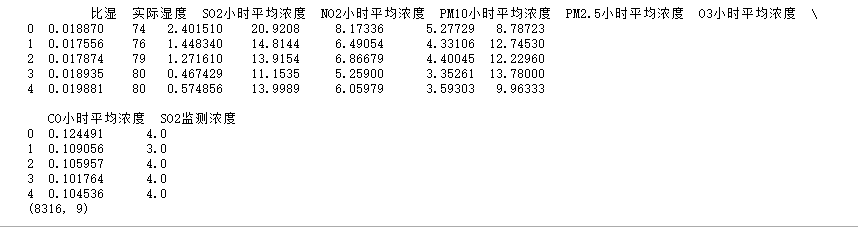4.归一化处理，去除量纲差别

#首先归一化，再移位处理，得到训练样本
scaler = MinMaxScaler(feature_range=(0, 1))
scaledData1 = scaler.fit_transform(data1)
print(scaledData1.shape)



5.使用time_series_to_supervised函数生成适用于监督学习的 DataFrame

n_steps_in =72 #历史时间长度
n_steps_out=1#预测时间长度
processedData1 = time_series_to_supervised(scaledData1,n_steps_in,n_steps_out)



可以打印一下看看生成的dataframe：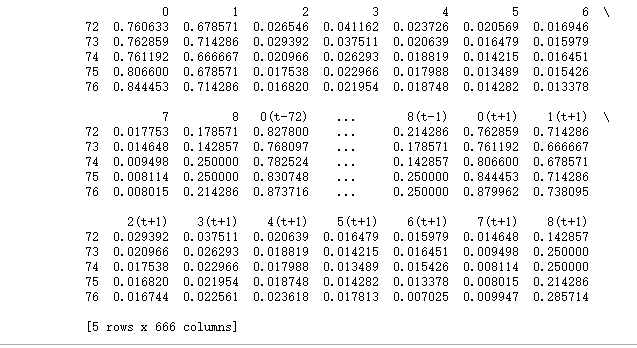6.划分特征与标签

data_x = processedData1.loc[:,'0(t-72)':'8(t-1)']
data_y = processedData1.loc[:,'0':'8']



如果你只是预测9个特征中的其中1个或几个特征的值，在data_y这里做相应调整。比如，我只想预测“So2监测浓度”（第九列）的数据，那第二行应改为：

data_y = processedData1.loc[:,'8']


值得注意的是：若我们对data_y进行修改，比如我们仅预测特征最后一列时，后面对模型预测结果进行反归一化会报出维数不匹配的问题。这是因为我们一开始归一化fit_transform()的是整个数据集，包含了所有的特征列，而后续我们想反归一化时，仅是对我们想要预测的值，即单一特征列，进行反归一化，所以会出现维数不匹配的情况。建议可以预测所有特征列，最后结果仅提取你想要的预测列结果即可，像我下面仅使用了预测结果的最后一列，即y_pre[:,8]一样。或者sklearn.preprocessing.MinMaxScaler.inverse_transform()是否可以仅对部分列反归一化，从而消除维数不匹配问题（我在网上没有搜到相关用法，有知道的可以在评论区补充哦~）
7.划分训练集和测试集

train_X1,test_X1, train_y, test_y = train_test_split(data_x.values, data_y.values, test_size=0.4, random_state=343)
# reshape input to be 3D [samples, timesteps, features]
train_X = train_X1.reshape((train_X1.shape, n_steps_in, scaledData1.shape))
test_X = test_X1.reshape((test_X1.shape, n_steps_in, scaledData1.shape))
print(train_X.shape, train_y.shape, test_X.shape, test_y.shape)


使用train_test_split函数划分训练集和测试集，测试集的比重是40%。
然后将train_X1、test_X1进行一个升维，变成三维，维数分别是[samples,timesteps,features]。打印一下他们的shape：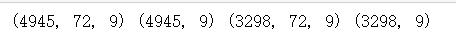8.构建模型


# design network
model = Sequential()
#input_shape千万不要写错！，第一个参数可以更改
model.add(LSTM(64, return_sequences=False))  # returns a sequence of vectors of dimension 32
print(model.summary())
# fit network
history = model.fit(train_X, train_y, epochs=30, batch_size=64, validation_data=(test_X, test_y), verbose=2,
shuffle=False)
# plot history
plt.plot(history.history['loss'], label='train')
plt.plot(history.history['val_loss'], label='test')
plt.legend()
plt.show()


输出结果：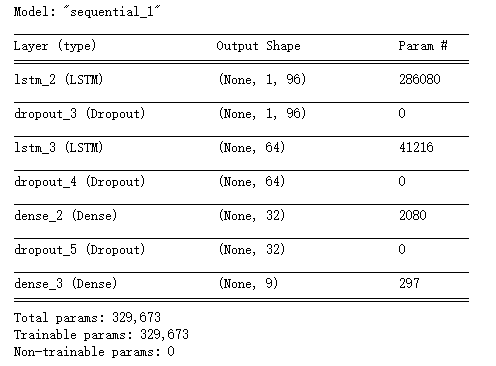loss变化图：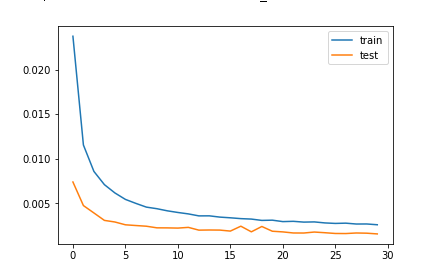9.使用模型

 # 预测
yhat = model.predict(test_X)
# 反归一化
inv_forecast_y = scaler.inverse_transform(yhat)
inv_test_y = scaler.inverse_transform(test_y)



用predict方法进行预测。因为之前我们对数据进行了归一化处理，所以要想获得真实的预测值，需要反归一化。
9.计算均方误差与画图

# 计算均方根误差
rmse = sqrt(mean_squared_error(inv_test_y[:,8], inv_forecast_y[:,8]))
print('Test RMSE: %.3f' % rmse)
#画图
plt.figure(figsize=(16,8))
plt.plot(inv_test_y[:,8], label='true')
plt.plot(inv_forecast_y[:,8], label='pre')
plt.legend()
plt.show()


这里我只做了对第九列“So2监测浓度”的预测值与实际值的均方误差与图像对比，因为数据太多，只展示了前300行的数据。从图片看拟合程度还是不错的。
请注意，图片展示的是测试集中的部分数据，因为我们一开始将训练集和测试集打乱顺序划分的，所以下图展示数据并非时序。如果想要获得时序数据，有两种方法：1.划分训练集和测试集的时候不打乱顺序，按顺序划分，比如前80%为训练集，后20%为测试集；2.使用data_x输入模型获得的预测值与data_y比较（注意data_x输入模型时需要升维），这个数据可以用来画图展示，但不能用来得到模型的rmse或mse等评价值，因为包含训练集的数据，会放大模型的效果。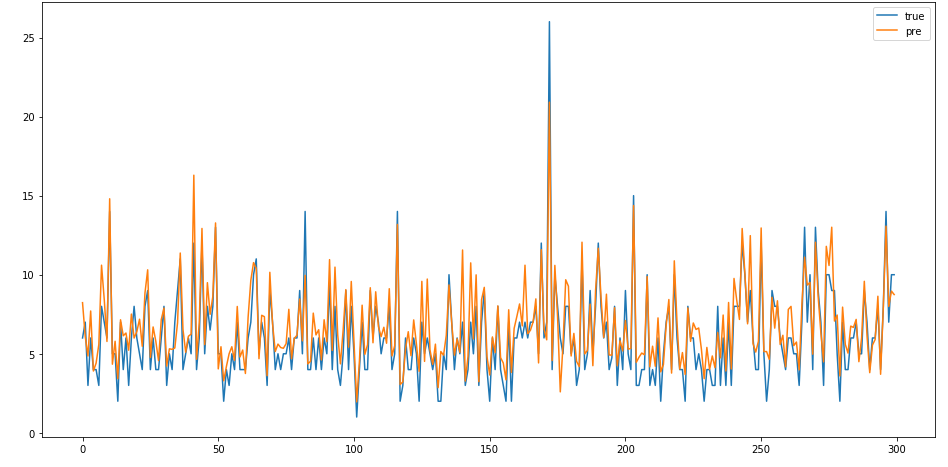以上模型就训练好可以使用了。
10.使用模型

#预测数据
#预测数据
data_x1= data_x.values[-1:,:]
print(data_x.shape)
data_x1= data_x1.reshape((data_x1.shape, n_steps_in, scaledData1.shape))

yhat_pre= model.predict(data_x1)

# 逆转预测值
yhat_pre= scaler.inverse_transform(yhat_pre)
print(yhat_pre)


使用表中给出的数据预测下一时刻的9个特征的值，结果如下：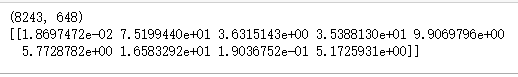这里一定要记得data_x1需要升维才能喂给LSTM！还有，我这里使用的data_x是已经经过前面time_series_to_supervised函数处理过的，如果你换了个新的数据，记得要先处理一下，把它变成这种步长内的数据横向拼接的形式。

全部代码：

from math import sqrt
from numpy import concatenate
from matplotlib import pyplot
import pandas as pd
# from pandas import DataFrame
# from pandas import concat
from sklearn.preprocessing import MinMaxScaler
from sklearn.preprocessing import LabelEncoder
from sklearn.metrics import mean_squared_error
from tensorflow.keras import Sequential
# from keras import models
from tensorflow.keras.layers import Dense
from tensorflow.keras.layers import LSTM
from tensorflow.keras.layers import Dropout
from sklearn.model_selection import train_test_split
import matplotlib.pyplot as plt
import numpy as np

def time_series_to_supervised(data, n_in=1, n_out=1,dropnan=True):
"""
:param data:作为列表或2D NumPy数组的观察序列。需要。
:param n_in:作为输入的滞后观察数（X）。值可以在[1..len（数据）]之间可选。默认为1。
:param n_out:作为输出的观测数量（y）。值可以在[0..len（数据）]之间。可选的。默认为1。
:param dropnan:Boolean是否删除具有NaN值的行。可选的。默认为True。
:return:
"""
n_vars = 1 if type(data) is list else data.shape
df = pd.DataFrame(data)
origNames = df.columns
cols, names = list(), list()
cols.append(df.shift(0))
names += [('%s' % origNames[j]) for j in range(n_vars)]
n_in = max(0, n_in)
for i in range(n_in, 0, -1):
time = '(t-%d)' % i
cols.append(df.shift(i))
names += [('%s%s' % (origNames[j], time)) for j in range(n_vars)]
n_out = max(n_out, 0)
for i in range(1, n_out+1):
time = '(t+%d)' % i
cols.append(df.shift(-i))
names += [('%s%s' % (origNames[j], time)) for j in range(n_vars)]
agg = pd.concat(cols, axis=1)
agg.columns = names
if dropnan:
agg.dropna(inplace=True)
return agg

# 加载数据
path1 = r"C:\data.xlsx"
data1 = datas1.iloc[:,np.r_[3,23,16:22,27]]
data1=data1.interpolate()
values1 = data1.values
#首先归一化，再移位处理，得到训练样本
scaler = MinMaxScaler(feature_range=(0, 1))
scaledData1 = scaler.fit_transform(data1)

n_steps_in =72 #历史时间长度
n_steps_out=1#预测时间长度
processedData1 = time_series_to_supervised(scaledData1,n_steps_in,n_steps_out)

data_x = processedData1.loc[:,'0(t-72)':'8(t-1)']
data_y = processedData1.loc[:,'0':'8']

train_X1,test_X1, train_y, test_y = train_test_split(data_x.values, data_y.values, test_size=0.4, random_state=343)
# reshape input to be 3D [samples, timesteps, features]
train_X = train_X1.reshape((train_X1.shape, n_steps_in, scaledData1.shape))
test_X = test_X1.reshape((test_X1.shape, n_steps_in, scaledData1.shape))
print(train_X.shape, train_y.shape, test_X.shape, test_y.shape)

# design network
model = Sequential()
model.add(LSTM(64, return_sequences=False))  # returns a sequence of vectors of dimension 32
print(model.summary())
# fit network
history = model.fit(train_X, train_y, epochs=30, batch_size=64, validation_data=(test_X, test_y), verbose=2,
shuffle=False)
# plot history
plt.plot(history.history['loss'], label='train')
plt.plot(history.history['val_loss'], label='test')
plt.legend()
plt.show()

# 预测
yhat = model.predict(test_X)
# 逆转预测值
print(yhat[:,8])
inv_forecast_y = scaler.inverse_transform(yhat)
# 逆转实际值
inv_test_y = scaler.inverse_transform(test_y)
# 计算均方根误差
rmse = sqrt(mean_squared_error(inv_test_y[:,8], inv_forecast_y[:,8]))
print('Test RMSE: %.3f' % rmse)
#画图
plt.figure(figsize=(16,8))
plt.plot(inv_test_y[0:300,8], label='true')
plt.plot(inv_forecast_y[0:300,8], label='pre')
plt.legend()
plt.show()

展开全文lstm 深度学习 神经网络 python keras
• tensorflow下用LSTM网络进行时间序列预测，实时多变量预测以及对于未来数据的单变量预测。
• 【Matlab源码】基于LSTM时间序列预测及一元和多元数据超参数调整的贝叶斯优化算法matlab
• TensorFlow版本：1.9.0 Keras版本：2.0.2 我的博客： :
• 使用pytorch搭建的简单的LSTM多变量多输出时间序列预测的使用例。 生成了多个以sinx、cosx、tanx构成的序列，使用[i:i+50]的数据预测[i+51]的数据。x是步长为0.1的等差数列 作者初学时用来当说明文档使用，程序适合...
• ## pytorch LSTM时间序列预测

千次阅读 热门讨论 2021-12-02 11:28:49
pytorch LSTM 时间序列预测

### pytorch LSTM 时间序列预测

#%%
import pandas as pd
import numpy as np
import torch
from torch import nn
from torch.nn import functional as F
import torchkeras
from plotly import graph_objects as go
from sklearn.preprocessing import MinMaxScaler

#%%
# 导入数据
# 数据下载：https://www.kaggle.com/kankanashukla/champagne-data
# 下载地址2：https://gitee.com/jejune/Datasets/blob/master/champagne.csv

Month   Sales
1964-01 2815
1964-02	2672
1964-03	2755
1964-04	2721
1964-05	2946

#%%
# 数据预览
fig = go.Figure()
fig.show()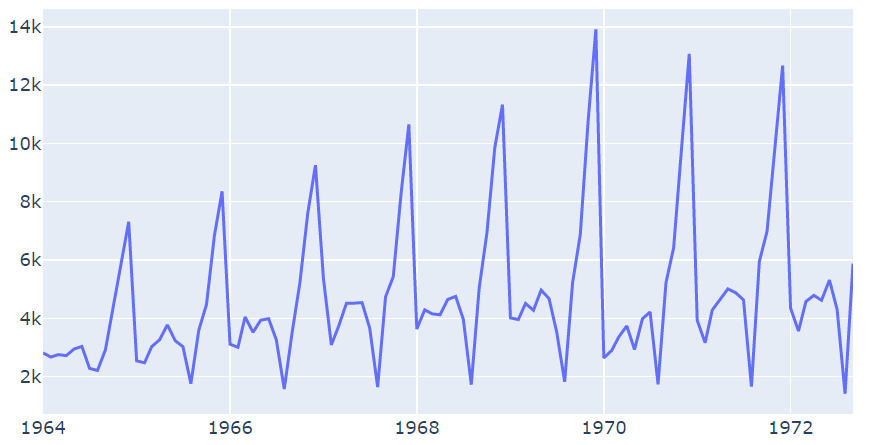#%%
# 数据处理
# 归一化 [0, 1]
scaler = MinMaxScaler()
predict_field = 'Scaler'
df[predict_field] = scaler.fit_transform(df['Sales'].values.reshape(-1, 1))

Month	Sales	Scaler
1964-01	2815    0.112133
1964-02	2672	0.100696
1964-03	2755	0.107334
1964-04	2721	0.104615
1964-05	2946	0.122611

#%%
def create_dataset(data:list, time_step: int):
arr_x, arr_y = [], []
for i in range(len(data) - time_step - 1):
x = data[i: i + time_step]
y = data[i + time_step]
arr_x.append(x)
arr_y.append(y)
return np.array(arr_x), np.array(arr_y)

time_step = 8
X, Y = create_dataset(df[predict_field].values, time_step)

#%%
# cuda
device = torch.device("cuda:0" if torch.cuda.is_available() else "cpu")
# 转化成 tensor->(batch_size, seq_len, feature_size)
X = torch.tensor(X.reshape(-1, time_step, 1), dtype=torch.float).to(device)
Y = torch.tensor(Y.reshape(-1, 1, 1), dtype=torch.float).to(device)
print('Total datasets: ', X.shape, '-->', Y.shape)

# 划分数据
split_ratio = 0.8
len_train = int(X.shape * split_ratio)
X_train, Y_train = X[:len_train, :, :], Y[:len_train, :, :]
print('Train datasets: ', X_train.shape, '-->', Y_train.shape)

Total datasets:  torch.Size([96, 8, 1]) --> torch.Size([96, 1, 1])
Train datasets:  torch.Size([76, 8, 1]) --> torch.Size([76, 1, 1])

#%%
# 构建迭代器
batch_size = 10
ds = TensorDataset(X, Y)
ds_train = TensorDataset(X_train, Y_train)
# 查看第一个batch
x, y = next(iter(dl_train))
print(x.shape)
print(y.shape)

torch.Size([10, 8, 1])
torch.Size([10, 1, 1])

#%%
# 定义模型
class Net(nn.Module):
def __init__(self):
super(Net, self).__init__()
self.lstm = nn.LSTM(input_size=1, hidden_size=6, num_layers=3, batch_first=True)
self.fc = nn.Linear(in_features=6, out_features=1)

def forward(self, x):
# x is input, size (batch_size, seq_len, input_size)
x, _ = self.lstm(x)
# x is output, size (batch_size, seq_len, hidden_size)
x = x[:, -1, :]
x = self.fc(x)
x = x.view(-1, 1, 1)
return x

#%%
# torchkeras API 训练方式
model = torchkeras.Model(Net())
model.summary(input_shape=(time_step, 1))
dfhistory = model.fit(epochs=50, dl_train=dl_train, log_step_freq=20)


----------------------------------------------------------------
Layer (type)               Output Shape         Param #
================================================================
LSTM-1                 [-1, 8, 6]             888
Linear-2                    [-1, 1]               7
================================================================
Total params: 895
Trainable params: 895
Non-trainable params: 0
----------------------------------------------------------------
Input size (MB): 0.000031
Forward/backward pass size (MB): 0.000374
Params size (MB): 0.003414
Estimated Total Size (MB): 0.003819
----------------------------------------------------------------
Start Training ...

================================================================================2022-03-28 12:21:58

+-------+-------+
| epoch |  loss |
+-------+-------+
|   1   | 0.045 |
+-------+-------+

================================================================================2022-03-28 12:21:59

+-------+-------+
| epoch |  loss |
+-------+-------+
|   2   | 0.054 |
+-------+-------+

...

+-------+-------+
| epoch |  loss |
+-------+-------+
|   50  | 0.003 |
+-------+-------+

================================================================================2022-03-28 12:22:00
Finished Training...

#%%
# 模型评估
fig = go.Figure()
fig.show()#%%
# 预测验证预览
y_true = Y.cpu().numpy().squeeze()
y_pred = model.predict(dl).detach().cpu().numpy().squeeze()
fig = go.Figure()
fig.show()#%%
# 自定义训练方式
model = Net().to(device)
loss_function = nn.MSELoss()

def train_step(model,features, labels):
# 正向传播求损失
predictions = model.forward(features)
loss = loss_function(predictions, labels)
# 反向传播求梯度
loss.backward()
# 参数更新
optimizer.step()
return loss.item()

# 测试一个batch
features, labels = next(iter(dl_train))
loss = train_step(model, features, labels)
loss

0.016526855528354645

#%%
# 训练模型
def train_model(model, epochs):
for epoch  in range(1, epochs+1):
list_loss = []
for features, labels in dl_train:
lossi = train_step(model,features, labels)
list_loss.append(lossi)
loss = np.mean(list_loss)
if epoch % 10 == 0:
print('epoch={} | loss={} '.format(epoch,loss))

train_model(model, 50)

epoch=10 | loss=0.047142161638475955
epoch=20 | loss=0.03120349021628499
epoch=30 | loss=0.009641124575864524
epoch=40 | loss=0.002635095050209202
epoch=50 | loss=0.0018647939796210267

#%%
# 预测验证预览
y_pred = model.forward(X).detach().cpu().numpy().squeeze()
fig = go.Figure()
fig.show()展开全文pytorch lstm python
• 基于Keras的多元时间预测，关于空气污染，使用LSTM模型。
•多变量时间序列 LSTM keras
• 前三篇文章，讨论了单变量、多变量和多步时间序列预测。对于不同的问题，可以使用不同类型的LSTM模型，例如Vanilla、Stacked、Bidirectional、CNN-LSTM、Conv LSTM模型。这也适用于涉及多变量和多时间步预测的时间...深度学习 人工智能 神经网络 tensorflow lstm
• 完整代码已上传我的资源：【LSTM时间序列数据】基于matlab LSTM时间序列数据预测【含Matlab源码 1949期】获取代码方式2： 通过订阅紫极神光博客付费专栏，凭支付凭证，私信博主，可获得此代码。备注： 订阅紫极神光...matlab lstm 开发语言
• 预测模型用于许多不同的领域和应用程序。...在此博客文章中，我们想展示如何通过自行车共享案例研究将**基于长期记忆（LSTM）**的RNN用于多变量时间序列预测，在该案例中，我们基于多个输入功能来预测自行车的需求大数据 神经网络 机器学习 人工智能 python
• 提供一种适用于初中级学者的时间序列预测模型 并且十分的有效好用 数据介绍 该数据集是一个污染数据集，我们需要用该多维时间序列去预测pollution这个维度，采用80%作为训练集，20%作为测试集。 模型实现 模型使用...lstm 深度学习 keras 时间序列
• 总之，都是把时间序列，转化成监督学习，然后利用监督学习的算法进行预测，长短记忆网络还是蛮好用的，只是需要提前安装TensorFlow和keras from numpy import nan from numpy import isnan from pandas import ...
• 大家好，今天我给大家详细分享一篇使用 LSTM 进行端到端时间序列预测的实战项目，本文内容较长，欢迎收藏、点赞、关注。文末给出完整代码。 推荐文章 有人把吴恩达老师的机器学习和深度学习做成了中文版 上瘾了...lstm 深度学习 人工智能
• 根据历史数据进行时间序列建模，并且进行预测未来。 我的数据是time列 和 price列 的dataframe。从2007~2021.02.28的数据。 我做的事情是 预测未来的price，注意！不是测试集上的！因为对于时间序列来说，测试集上的...深度学习 时间序列 python LSTM
• 本节将介绍另一种常用的门控循环神经网络：长短期记忆（long short-term memory，LSTM）。它 比门控循环单元的结构稍微复杂一点。1.1、数据集和问题定义import torch import torch.nn as nn import seaborn as sns ...CNN做时间序列预测
• 时间序列预测 | Python实现LSTM多变量时间序列数据预测 目录时间序列预测 | Python实现LSTM多变量时间序列数据预测基本介绍程序设计参考资料 基本介绍 时间序列分析（Time-Series Analysis）是指将原来的销售分解为...lstm python 深度学习 时序模型
• 注重多元时间序列的LSTM自动编码器 该存储库包含用于多变量时间序列预测的自动编码器。 它具有描述的两种注意力机制，并且受启发。下载和依赖项要克隆存储库，请运行： git clone ...
• 之前我们已经在配水管网中使用了不同的用水系数乘子，本次我们想使用LSTM算法来预测节点用水量，压力的时间序列。 按照特征工程与实际的专业知识，我们使用滑动窗口的平移输入，以7天为一个周期，输入7天的节点流量...
• 使用LSTM和自动阈值检测时间序列数据中的异常 Telemanom采用使用香草LSTMs / 识别多元传感器数据中的异常。 LSTM经过训练，可以使用编码的命令信息和先前的遥测值来学习正常的系统行为。 预测会在每个时间步生成，...
• 此示例中，神经网络用于使用2011年4月至2013年2月期间的数据预测都柏林市议会公民办公室的能源消耗。 每日数据是通过总计每天提供...当涉及到这项任务时，传统的神经网络不足，在这方面，LSTM将用于预测这种情况下...Python
• 时间序列预测 | Python实现Prophet、ARIMA、LSTM时间序列数据预测 目录时间序列预测 | Python实现Prophet、ARIMA、LSTM时间序列数据预测数据描述特征工程构建模型程序设计致谢 数据描述 首先读取数据，并找到对应的...python 时序模型 机器学习
•lstm 深度学习
• 本文介绍了如何在 Keras 深度学习库中搭建用于多变量时间序列预测LSTM 模型。 诸如长短期记忆（LSTM）循环神经网络的神经神经网络几乎可以无缝建模具备多个输入变量的问题。 这为时间序列预测带来极大益处，因为...
• 背景相关资料（1）Understanding LSTM Networks​colah.github.io（2）pytorch示例​machinelearningmastery.comLSTM解读LSTM示意图上图是LSTM相当经典的示意图了，上图中有三门一个细胞状态：信息的前向传播满足： ...CNN做时间序列预测
• 今天给大家分享一波使用 LSTM 进行端到端时间序列预测的完整代码和详细解释。我们先来了解两个主题：什么是时间序列分析？什么是 LSTM时间序列分析：时间序列表示基于时间顺序的一系列数据。它可以是秒、分钟、...python 机器学习 人工智能 深度学习 java
• 来源：DeepHub IMBA使用 LSTM 进行端到端时间序列预测的完整代码和详细解释。我们先来了解两个主题：什么是时间序列分析？什么是 LSTM时间序列分析：时间序列表示基于时间顺序...算法 python 机器学习 人工智能 深度学习...